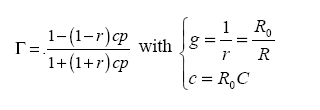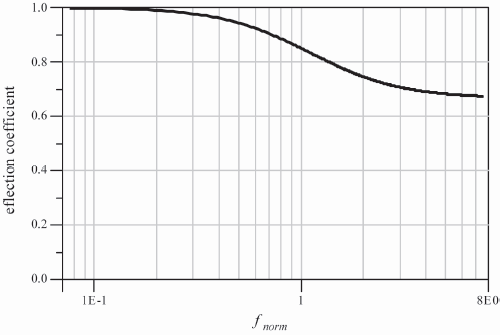Appendix 5

Wideband Impedance Matching: Dissipative Two-Port Networks

We will now detail the calculations relative to the wideband matching for two types of circuit characteristics of the transistors: the series-RC and the parallel-RC circuits.

# A5.1. Series-RC circuit

Consider a series-RC-type circuit as shown in Figure A5.1.The reflection coefficient normalized from reference impedance R0 is written as:A normalized frequency can be defined as:The variation as a function of the normalized frequencyis shown in Figure A5.2.

Figure A5.2. Module of the series-RC-type reflection coefficientThe low-frequency matching is obtained thanks to parallel resistance (Figure A5.3).

Figure A5.3. Series-RC resistive matchingThe reflection coefficient is written as:

Matching is achieved with gl = l, that is

Figure A5.4 shows variation as a function of ...

Get Design of Microwave Active Devices now with the O’Reilly learning platform.

O’Reilly members experience books, live events, courses curated by job role, and more from O’Reilly and nearly 200 top publishers.